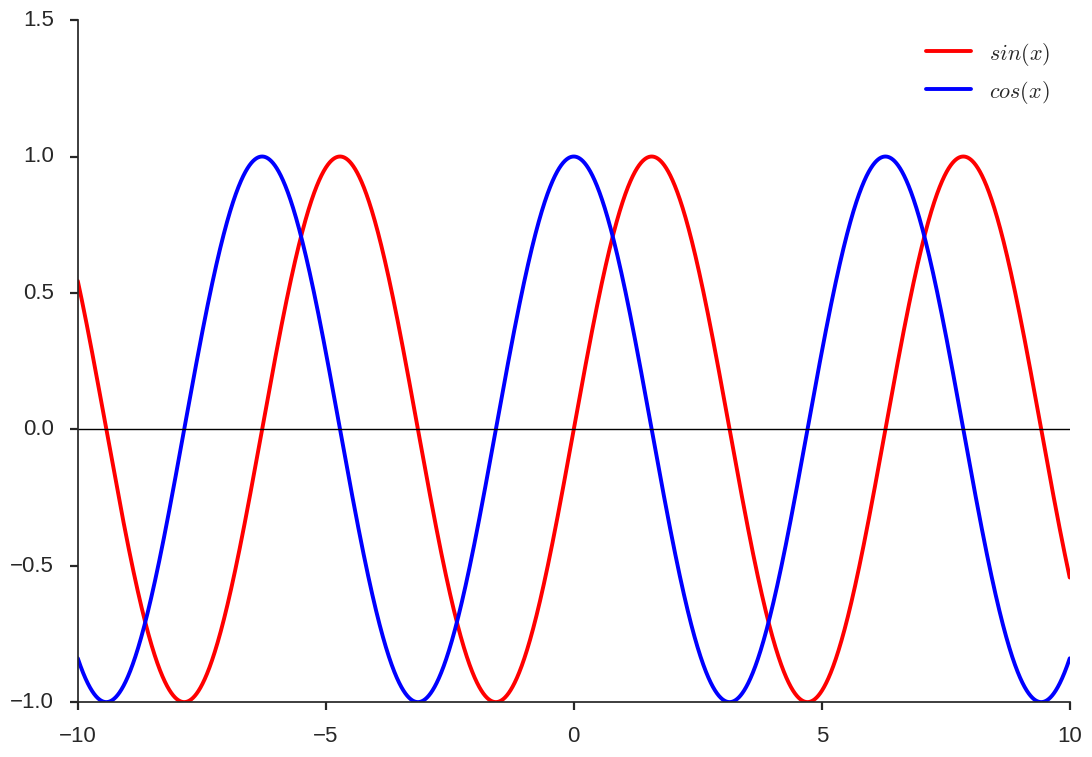# Getting started with pelican

Posted on 08/11/2016 in posts python firsts

# First plot using pelican¶

I am trying this new format for blogging using jupyter notebooks and pelican library. The motivation is to easily share my experiments using notebooks and write accompanying thoughts.

For this post I will test if the matplotlib plotting and latex equations work. I will simply be plotting the following 2 equations.

$x \in [-1,1]$

$y = sin(x)$

$y_1 = cos(x)$

In :
%matplotlib inline

import numpy as np
import pandas as pd

import matplotlib.pyplot as plt
import seaborn as sns

sns.set_context("poster")
sns.set_style("ticks")

In :
x = np.arange(-10,10, 0.001)
y = np.sin(x)
y1 = np.cos(x)
plt.plot(x,y, color="r", label="$sin(x)$")
plt.plot(x,y1, color="b", label="$cos(x)$")
plt.axhline(y=0, color="k", lw=1)
plt.ylim([-1,1.5])
plt.legend()
sns.despine()In :
df = pd.DataFrame({"x": x, "y": y, "y1": y1})

Out:
x y y1
0 -10.000 0.544021 -0.839072
1 -9.999 0.543182 -0.839615
2 -9.998 0.542342 -0.840158
3 -9.997 0.541501 -0.840700
4 -9.996 0.540660 -0.841241

So, everything works just fine. Let us try to deploy this to my github page.

In [ ]: